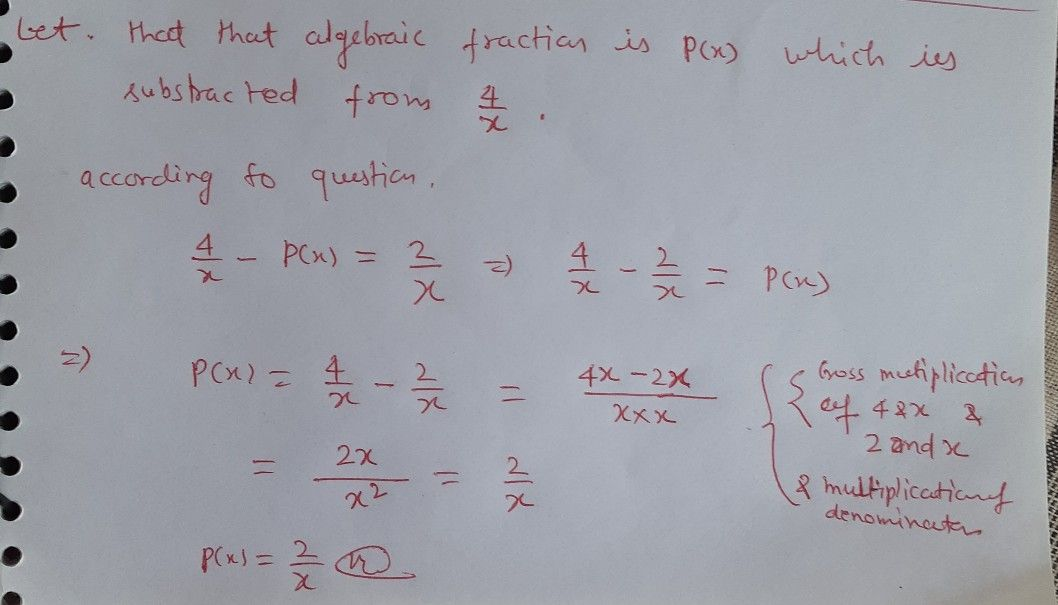Symbol
Problem$2$ When a rational algebraic expression is subtracted from The result is What is the other rational algebraic expression? $b\dfrac {x} {x-3}$ $2$ $c_{x}$ $a$ $\dfrac {2} {\bar{x-} 1}$
7th-9th grade
Algebra
Search count: 106
Question content
with solution pls
SolutionQanda teacher - satya265